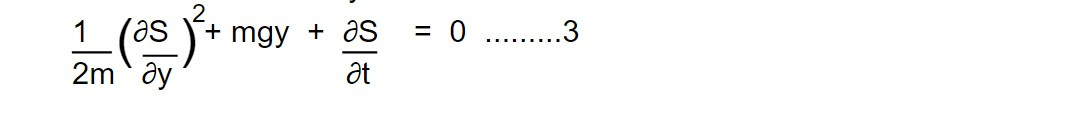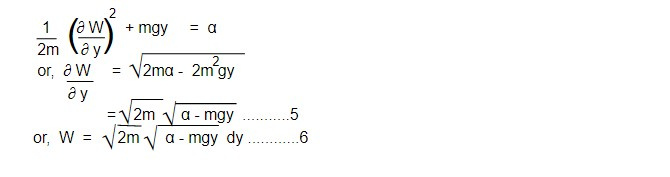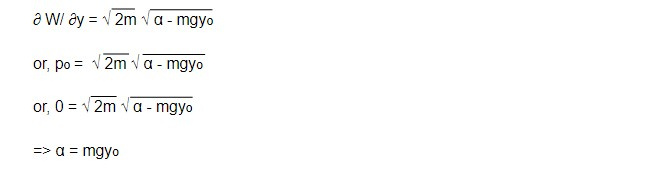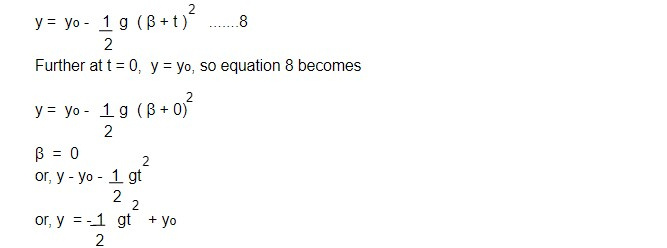# Freely falling particle using the H-J method

To determine the motion of a particle falling vertically in a uniform gravitational field, the Hamiltonian is given by

H = T + Vwhere y axis is taken as vertical [ i.e y = q]

Putting p = ∂s/∂y in eq 1 we getThen the H-J equation, H + ∂s/∂t = 0 becomes,Writing the solution of equation 3 in the form,

ρ (y, α, t)= W (y, α) - αt .........4

Then equation 3 becomes,Using eq 6 in eq4, then we getThen, β' = ∂s/∂αLet the initial conditions be such that at t= 0 (particle is at rest)

y = yo (initial displacement)

Then from equation 5, we haveThen eq 7 becomeswhich is the required equation of path of a particle falling vertically under the action of gravity.

This note is taken from Classical Mechanics, MSC physics, Nepal.

This note is a part of the Physics Repository.